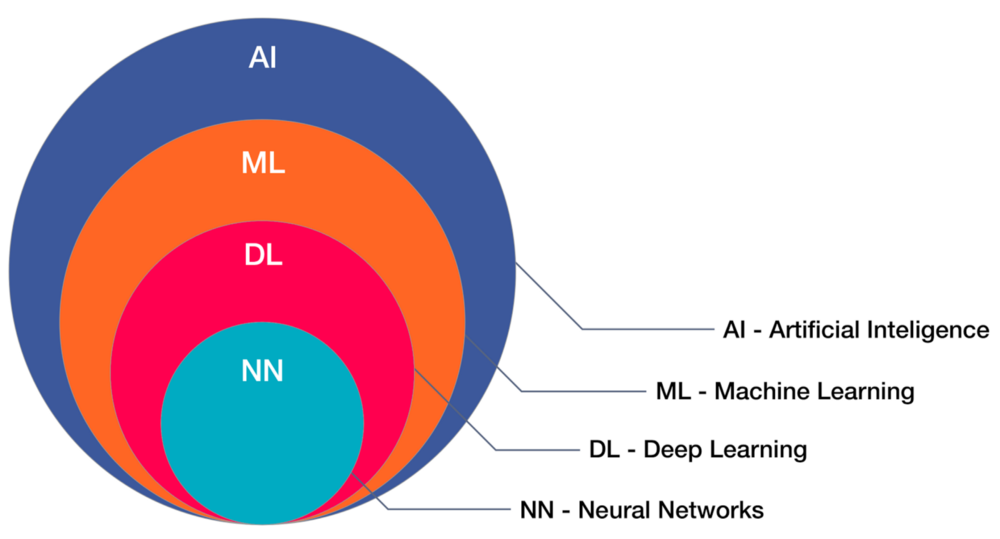3 views

Can you offer a concise explanation of the differences between Deep Learning and Traditional Machine Learning that utilize neural networks? How many levels are needed to make a neural network "deep"? Is this all just marketing hype?

by (108k points)

Here is a diagrammatic representation of relations between AI, ML, DL, Data Science:As you can see in the diagram, AI is the main head of all the other technologies and at the same time, all technologies are co-related to each other. ML, DL, NN all are the sub-parts of AI.

Deep learning requires an NN (neural network) having multiple layers in which each layer doing mathematical transformations and feeding into the next layer. The output from the last layer is the decision of the network for a given input. The layers that are present between the input and output layers are known as the hidden layers.

A neural network is a massive collection of perceptrons that are interconnected in layers. The weights and bias of each perceptron in the network depend upon the nature of the output decision of the entire network. In a perfectly tuned neural network, all the values of weights and bias of all the perceptron are such that the output decision is always correct (as expected) for all possible inputs.

To learn more about Deep learning and machine learning, you can enroll yourself in a Machine learning course.